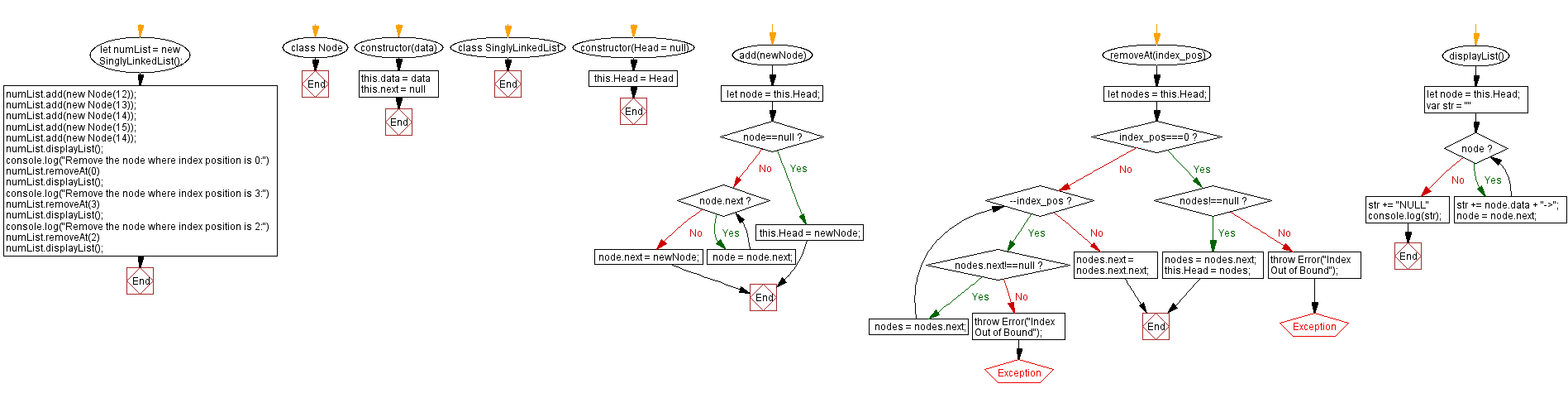# JavaScript Exercises: Remove a node at the specified index in a singly linked list

## JavaScript Singly Linked List: Exercise-11 with Solution

Write a JavaScript program that removes the node from the singly linked list at the specified index.

Sample Solution:

JavaScript Code:

``````class Node {
constructor(data) {
this.data = data
this.next = null
}
}
}
if(node==null){
return;
}
while (node.next) {
node = node.next;
}
node.next = newNode;
}
removeAt(index_pos){
if(index_pos===0) {
if(nodes!==null)
{
nodes = nodes.next;
}
else
throw Error("Index Out of Bound");

return;
}
while(--index_pos){
if(nodes.next!==null)
nodes = nodes.next;
else
throw Error("Index Out of Bound");
}
nodes.next = nodes.next.next;
}
displayList(){
var str = ""
while (node) {
str += node.data + "->";
node = node.next;
}
str += "NULL"
console.log(str);
}
}
numList.displayList();
console.log("Remove the node where index position is 0:")
numList.removeAt(0)
numList.displayList();
console.log("Remove the node where index position is 3:")
numList.removeAt(3)
numList.displayList();
console.log("Remove the node where index position is 2:")
numList.removeAt(2)
numList.displayList();
```
```

Sample Output:

```12->13->14->15->14->NULL
Remove the node where index position is 0:
13->14->15->14->NULL
Remove the node where index position is 3:
13->14->15->NULL
Remove the node where index position is 2:
13->14->NULL
```

Flowchart:Live Demo:

See the Pen javascript-singly-linked-list-exercise-11 by w3resource (@w3resource) on CodePen.

Improve this sample solution and post your code through Disqus

What is the difficulty level of this exercise?

Test your Programming skills with w3resource's quiz.

﻿

## JavaScript: Tips of the Day

Shorten an array using its length property

A great way of shortening an array is by redefining its length property.

```let array = [0, 1, 2, 3, 4, 5, 6, 6, 8, 9]
array.length = 4
// Result: [0, 1, 2, 3]
```

Important to know though is that this is a destructive way of changing the array. This means you lose all the other values that used to be in the array.

Ref: https://bit.ly/2LBj213